Pure and Applied Mathematics Journal
Volume 5, Issue 5, October 2016, Pages: 155-159

A Note on Strict Commutativity of a Monoidal Product

Youngsoo Kim

Department of Mathematics, Tuskegee University, Tuskegee, USA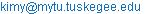Youngsoo Kim. A Note on Strict Commutativity of a Monoidal Product. Pure and Applied Mathematics Journal. Vol. 5, No. 5, 2016, pp. 155-159. doi: 10.11648/j.pamj.20160505.13

Received: August 25, 2016; Accepted: September 5, 2016; Published: September 21, 2016

Abstract: It is well known that a monoidal category is (monoidally) equivalent to a strict monoidal category that is a monoidal category with a strictly associative product. In this article, we discuss strict commutativity and prove a necessary and sufficient condition for a symmetric monoidal category to be equivalent to another symmetric monoidal category with a strictly commutative monoidal product.

Keywords: Symmetric Monoidal Category, Strict Commutativity, Monoidal Product

Contents

1. Introduction

A monoidal category is a category C with a monoidal product , unit object I, associator α, left unitor λ, and right unitor ρ satisfying commutativity of coherence diagrams describing the associativity of and the unit properties of I [8, VII.1]. There is an abundance of examples of monoidal categories — sets with cartesian product, abelian groups with tensor product, etc.

A monoidal category C is a strict monoidal category if the natural isomorphisms α, λ, and ρ are identities. It implies the following equalities on objects as well as morphisms. For any objects A, B, and C, and for any morphisms f, g, and h,

(AB)⊗C = A(BC), IA = A, AI = A,

(fg)h = f⊗(gh), 1If = f, f1I = f.

Monoidal functors and monoidal natural transformations are the ones respecting monoidal structures. Mac Lane’s theorem says that every monoidal category is monoidally equivalent to a strict monoidal category [6,8,9]. In other words, for any monoidal category C, there exists a strict monoidal category D, monoidal functors F: CD and G: DC, and monoidal natural isomorphisms GF 1C and FG 1D. With Mac Lane’s theorem, we can treat monoidal products as if they are strictly associative. In the spirit of the theorem, there have been results in the direction of strictifying associativity of diverse algebraic structures [9,4,5].

A symmetric monoidal category is a monoidal category C with a natural isomorphism

σA,B: AB→BA

for each pair A,BC such that σA,B = σB,A−1 satisfying commutativity of more diagrams [8, XI.1] relating associativity and commutativity. A monoidal product is said to be strictly commutative if σA,B is the identity for every pair of objects A and B. This implies the following equalities on objects and morphisms. For any objects A and B, and for any morphisms f and g, AB = BA and fg = gf.

Weakening the symmetry condition leads to the definition of the braided monoidal category and it has applications to knot theory and the theory of quantum groups [6,3]. Weakening the condition even further leads to the weak braided monoidal categories, which have been studied in [1,10]. On the other hand, strict commutativity of a monoidal product leads to very desirable properties in many situations. So it is natural for people to try to construct a monoidal category with strictly commutative product that is equivalent to the original category they are working with. However, is not hard to see that strictifying commutativity is not always possible. See Proposition 1 for a necessary condition. This condition has been noted by several authors. See Section 3.19 in  for example. In this paper, we show that this necessary condition is also sufficient if one assumes the axiom of choice.

2. Basic Definitions

We state the following definitions from  for easy reference.

Definition 1. A monoidal category is a category C with a bifunctor ⊗: C × CC called the monoidal product, an object I called the unit, and natural isomorphisms

αA,B,C: (AB)CA(BC) (associator)

λA: IAA (left unitor)

ρA: AIA(right unitor)

such that the following diagrams commute for all objects A, B, C, and D in C.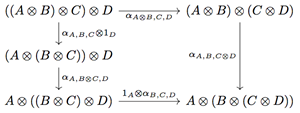(1)(2)

Definition 2. A symmetric monoidal category is a monoidal category with natural isomorphisms

σA,B: ABBA

such that for all objects A, B, and C, the following diagrams commute.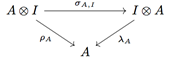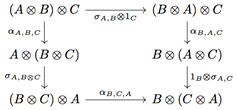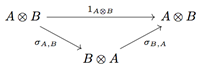(3)

A monoidal category or a symmetric monoidal category is called strict if α, λ, and ρ are all identities. A symmetric monoidal category is said to be strictly commutative if σ is the identity.

Definition 3. A monoidal functor between monoidal categories C and D is a functor F: CD with natural isomorphisms

φA,B: F(A)F(B) → F(AB)

and an isomorphism ε: JF(I) where J is the unit object of D such that the following diagrams commute.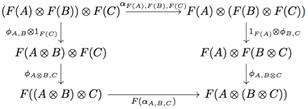(4)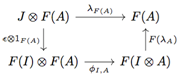(5)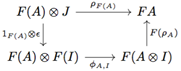Definition 4. A monoidal functor F: CD between symmetric monoidal categories is called symmetric if the following diagram commutes for every A, B C.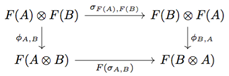(6)

Definition 5. Suppose (F,φ,ε) and (G,φ’,ε’) are (symmetric) monoidal functors CD between (symmetric) monoidal categories. A natural transformation γ: F G is called (symmetric) monoidal if the following diagrams commute for all A, B C.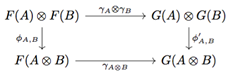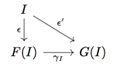3. Strict Commutativity

Proposition 1. Suppose C is a symmetric monoidal category that is symmetrically monoidally equivalent to a strictly commutative symmetric monoidal category. Then for any object A in C, the symmetry isomorphism σA,A: AA → AA must be the identity.

Proof. The natural isomorphism ξ: GF 1C gives the following commutative diagram.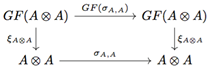(7)

The diagram (6) with A = B gives

φA,AσF(A),F(A) = F(σA,AA,A.

Since D is strictly commutative, σF(A),F(A) = 1. Therefore, φA,A = F(σA,A)φA,A, and after canceling the isomorphism φA,A, we get F(σA,A) = 1. Consequently, GF(σA,A) = 1. Now the diagram (7) gives

ξAA = σA,AξAA

and canceling the isomorphism ξAA, we get σA,A = 1.

The consequence of this proposition is that the commutativity of not every monoidal product can be strictified. In fact, the commutativity of many naturally arising monoidal products cannot be strictified as in the examples below.

Example 1. Consider (Set, ×), the category of sets with cartesian product. For a set A, the symmetry isomorphism σA,A is defined by σA,A(a1, a2) = (a2, a1). So σA,A is not the identity if A has more than one element. Therefore, × cannot be strictified to be strictly commutative.

Example 2. Consider (Vect(k), ), the category of vector spaces over a field k with tensor product. For a vector space V, the symmetry isomorphism σV,V is defined by σV,V(vivj) = vjvi. Suppose dimV > 1 and v1 and v2 are linearly independent. Then v1v2 is not equal to v2v1. Therefore, cannot be strictified to be strictly commutative.

Corollary 1. Suppose C is a symmetric monoidal category with only one object. Then C is equivalent to a strictly commutative symmetric monoidal category if and only if C is strictly commutative.

Proof. Suppose C is equivalent to a strictly commutative symmetric monoidal category. By Proposition 1, σA,A is the identity for the unique object A. Since it is the only symmetry morphism to be checked, C is strictly commutative. The converse is obvious.

The main theorem of the paper is the following. It says the converse of Proposition 1 is also true if objects of C can be totally orderd. If we assume axiom of choice and restrict our attention to a small category or Grothendieck universe, this assumption is satisfied.

Theorem 1. Let C be a symmetric monoidal category satisfying two conditions.

(1)  There is a total order on objects of C.

(2)  For all objects A in C, σA,A = 1AA.

Then C is symmetrically monoidally equivalent to a strictly commutative symmetric monoidal category.

To prove the theorem, we will introduce a new monoidal structure on C that is strictly commutative, then we will prove the equivalence of two monoidal structures. We define a new product as follows. For A, B C, define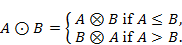For morphisms f: AC and g: BD, define fg byNext, define πA,B: ABAB by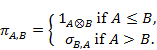Lemma 1. The following diagram commutes for any f: AC and g: BD.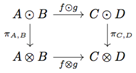Proof. This lemma follows from the property of σ, that is, σA,B = σB,A−1 for all A, B C. (See diagram (3).) We can easily check four cases individually.

We can say π is a natural isomorphism once we prove the next lemma.

Lemma 2. The new product is a bifunctor.

Proof. For the identity rule,

1A 1B = 1A1B = 1AB

by definition. The composition rule is proved using Lemma 1. For any

(f, g): (A, B) → (C, D)

and

(h,k): (C, D) → (E, F),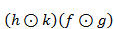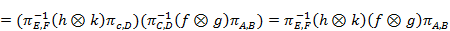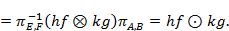Now we define new associator left and right unitors, and symmetry morphism. Define α’, λ’, ρ’, and σ’ in such a way that the following diagrams commute for all A, B, C in C. They are natural isomorphisms as compositions of natural isomorphisms.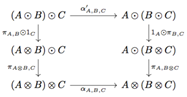(8)(9)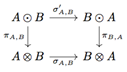(10)

Proposition 2. Under the assumptions of Theorem 1, the bifunctor together with the same unit object I, and natural isomorphisms α’, λ’, ρ’, and σ’ defined above gives C a strictly commutative symmetric monoidal structure.

Proof. Using the diagrams (8), (9), and (10), we can replace each object and morphism of the commutative diagrams (1) – (3) with the corresponding objects and morphisms in terms of . Another way to see the commutativity of such diagrams is by using Mac Lane’s coherence theorem [8, Theorem XI.1.1],  since π is defined in terms of σ and the identity only. Thus, C is a symmetric monoidal cagetory with . Now we will prove that σ’ is the identity. Consider three cases: A < B, A > B, and A = B.

Case 1: A < B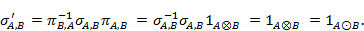Case 2: A > B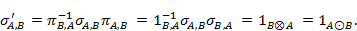Case 3: A = B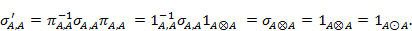Note that the second assumption of Theorem 1 is used in the last case.

Proof of Theorem 1. We have constructed a new monoidal structure on C with .

It remains to prove the equivalence of two monoidal structures. Define

F: (C, , I) → (C, , I)

to be the identity as a functor. Then define

φA,B: ABAB

to be πA,B and define ε = 1I. Then (F, φ, ε) is a symmetric monoidal functor because the diagrams (4), (5), and (6) translate into diagrams (8), (9), and (10), respectively. We define the inverse monoidal functor similarly. Let

G: (C,, I) → (C,, I)

be the identity as a functor, and define

ψA,B: ABAB

to be πA,B−1 and ε = 1I. Then (G, ψ, ε) is a symmetric monoidal functor. The diagrams (5) and (6) translate into (9) and (10), respectively. The diagram (4) translates into the following diagram.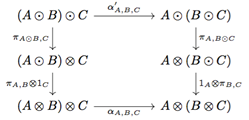The commutativity of this diagram follows from the commutativity of the diagram (8) and the equality of the following compositions of morphisms, which is the result of Mac Lane’s coherence theorem (or one may check individual cases).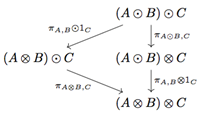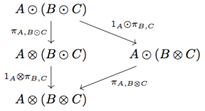Since (F, φ, ε) and (G, ψ, ε) are inverese to each other, two monoidal structures are isomorphic.

We can take one more step to achieve both strict associativity and strict commutativity as in the next theorem.

Theorem 2. Let C be a symmetric monoidal category satisfying two conditions.

(1)  There is a total order on objects of C.

(2)  For all objects A in C, σA,A =1AA.

Then C is symmetrically monoidally equivalent to a symmetric monoidal category with strictly associative and strictly commutative monoidal product.

Proof. We may assume C is strictly commutative by Theorem 1. We slightly modify Mac Lane’s construction of a strict symmetric monoidal category described in XI.3 in . Define a category D as follows. The objects are all finite strings

M = [A1,..., Ak]

in non-decreasing order A1 ··· Ak. Define MN for strings M and N to be the concatenation of the strings followed by rearranging objects in order. This product is strictly associative and strictly commutative on objects. The identity is the empty string . Then define a map F: DC by setting

F() = I,

F(M)=(...(A1A2)A3)...)Ak)

where the parentheses begin in front. Now define the morphisms of D by

MorD(M,N) = MorC(F(M),F(N)).

For morphisms f: MK and g: NL, define

fg: MNKL

to be the canonical map

F(MN) → F(M)F(N)

F(K)F(L) → F(KL).

There is no ambiguity in this definition for rearranging objects and morphisms in the non-decreasing order because C is already strictly commutative. This definition gives a symmetric monoidal structure on D, and F becomes a symmetric monoidal functor. The definition of the functor in the opposite direction and the rest of the proof follows mutatis mutandis as in Mac Lane’s proof of Theorem XI.3.1 in .

Example 3. Let C be a symmetric monoidal category. Suppose that the objects of C can be totally orderd and that for each pair of objects A, B in C, there is no more than one morphism from A to B. Then C is symmetrically monoidally equivalent to a strictly associative and strictly commutative symmetric monoidal category becase for any A in C, σA,A is an endomorphism, and it must be the unique morphism 1AA.

4. Conclusion

We have proved that, assuming the axiom of choice, the commutativity of the monoidal category in a symmetric monidal category can be strictified if and only if the symmetry maps σA,A for all objects A of the category are identities. This easily verifiable criterion resolves the strictification problem completely.

References

1. C. Balteanu, Z. Fiedorowicz, R. Schwänzl, and R. Vogt, Iterated monoidal categories, Adv. Math. 176 (2003), no. 2, 277–349. MR 1982884.
2. Mitya Boyarchenko, Associativity constraints in monoidal categories, http://math.uchicago.edu/~may/TQFT/Boyarchenko%20on%20associativity.pdf.
3. V. G. Drinfel′d, Quantum groups, Proceedings of the International Congress of Mathematicians, Vol. 1, 2 (Berkeley, Calif., 1986), Amer. Math. Soc., Providence, RI, 1987, pp. 798–820. MR 934283.
4. Bertrand J. Guillou, Strictification of categories weakly enriched in symmetric monoidal categories, Theory Appl. Categ. 24 (2010), No. 20, 564–579. MR 2770075.
5. Nick Gurski and Ang ́elica M. Osorno, Infinite loop spaces, and coherence for symmetric monoidal bicategories, Adv. Math. 246 (2013), 1–32. MR 3091798.
6. André Joyal and Ross Street, Braided tensor categories, Adv. Math. 102 (1993), no. 1, 20–78. MR 1250465.
7. Saunders Mac Lane, Natural associativity and commutativity, Rice Univ. Studies 49 (1963), no. 4, 28–46. MR 0170925.
8. Saunders Mac Lane, Categories for the working mathematician, second ed., Graduate Texts in Mathematics, vol. 5, Springer-Verlag, New York, 1998. MR 1712872.
9. Peter Schauenburg, Turning monoidal categories into strict ones, New York J. Math. 7 (2001), 257–265 (electronic). MR 1870871 (2003d:18013).
10. Mirjam Solberg, Weak braided monoidal categories and their homotopy colimits, Theory Appl. Categ. 30 (2015), 40–48. MR 3306878.

 Contents 1. 2. 3. 4.
Article ToolsAbstractPDF(847K)